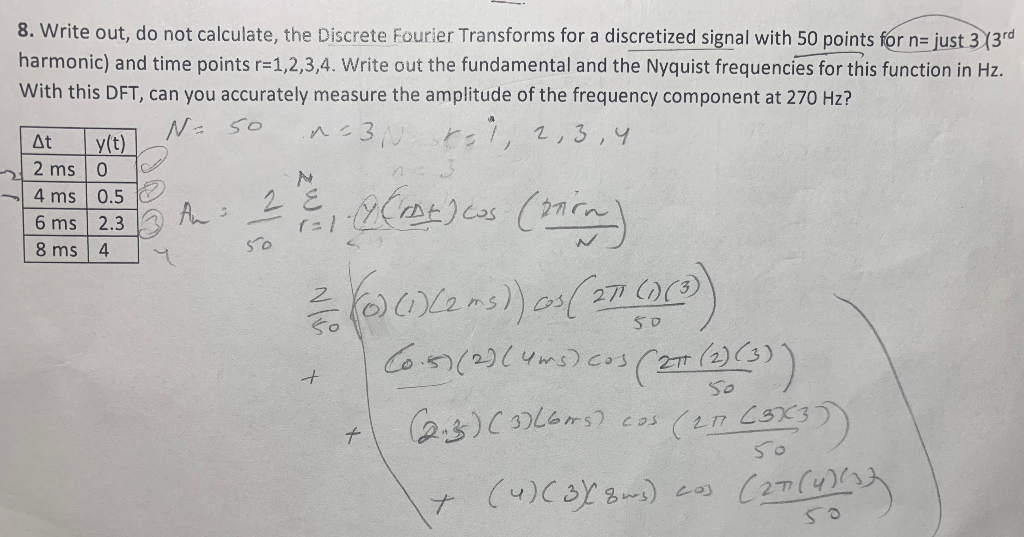Home / Answered Questions / Other / 8-write-out-do-not-calculate-the-discrete-fourier-transforms-for-a-discretized-signal-with-50-points-aw351

# (Solved): 8. Write Out, Do Not Calculate, The Discrete Fourier Transforms For A Discretized Signal With 50 Poi...8. Write out, do not calculate, the Discrete Fourier Transforms for a discretized signal with 50 points for n= just 3 13rd harmonic) and time points r=1,2,3,4. Write out the fundamental and the Nyquist frequencies for this function in Hz. With this DFT, can you accurately measure the amplitude of the frequency component at 270 Hz? V N-50 non ril, 2,3,4 At y(t) 2 mso 4 ms 0.5 6 ms 2.3 | 8ms 4 zW 6 ms 2:3 An 50 2 e-ACRAELos Crica 200162 ms)) (( 271 636) + 60-93(2)(ums).cos ( 27 (2) (3)) + 2.3.) (3) Loors) cos (27 (943) (4) (37 8urs) cos (27 (4) 50

We have an Answer from Expert